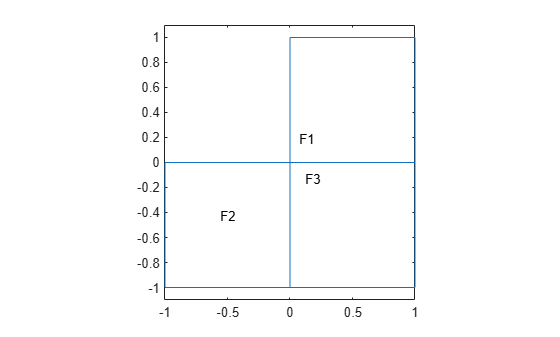Main Content

## View, Edit, and Delete Initial Conditions

### View Initial Conditions

A PDE model stores initial conditions in its `InitialConditions` property. Suppose `model` is the name of your model. Obtain the initial conditions:

`inits = model.InitialConditions;`

To see the active initial conditions assignment for a region, call the `findInitialConditions` function. For example, create a model and view the geometry.

```model = createpde(); geometryFromEdges(model,@lshapeg); pdegplot(model,'FaceLabels','on') ylim([-1.1,1.1]) axis equal```Specify constant initial conditions over all the regions in the model.

`setInitialConditions(model,2);`

Specify a different initial condition on each subregion.

```setInitialConditions(model,3,'Face',2); setInitialConditions(model,4,'Face',3);```

View the initial condition assignment for region 2.

```ics = model.InitialConditions; findInitialConditions(ics,'Face',2)```
```ans = GeometricInitialConditions with properties: RegionType: 'face' RegionID: 2 InitialValue: 3 InitialDerivative: [] ```

This shows the "last assignment wins" characteristic.

View the initial conditions assignment for region 1.

`findInitialConditions(ics,'Face',1)`
```ans = GeometricInitialConditions with properties: RegionType: 'face' RegionID: [1 2 3] InitialValue: 2 InitialDerivative: [] ```

The active initial conditions assignment for region 1 includes all three regions, though this assignment is no longer active for regions 2 and 3.

### Delete Existing Initial Conditions

To delete all the initial conditions in your PDE model, use `delete`. Suppose `model` is the name of your model. Remove all initial conditions from `model`.

`delete(model.InitialConditions)`

To delete specific initial conditions assignments, delete them from the `model.InitialConditions.InitialConditionAssignments` vector.

```icv = model.InitialConditions.InitialConditionAssignments; delete(icv(2))```

Tip

You do not need to delete initial conditions; you can override them by calling `setInitialConditions` again. However, deleting unused assignments can make your model smaller.

### Change an Initial Conditions Assignment

To change an initial conditions assignment, you need the initial conditions handle. To get the initial condition handle:

• Retain the handle when using `setInitialConditions`. For example,

`ics1 = setInitialConditions(model,2);`
• Obtain the handle using `findInitialConditions`. For example,

```ics = model.InitialConditions; ics1 = findInitialConditions(ics,'Face',2);```

You can change any property of the initial conditions handle. For example,

```ics1.RegionID = [1,3]; ics1.InitialValue = 2; ics1.InitialDerivative = @ut0fun;```

Note

Editing an existing assignment in this way does not change its priority. For example, if the active initial conditions in region `3` was assigned after `ics1`, then editing `ics1` to include region `3` does not make `ics1` the active initial condition in region `3`.

## Support평가판 신청